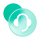## 1. 简介

### 1.3 预期效果## 2. 操作步骤

### 2.1 表单配置### 2.2 设置正确答案字段• 选择的答案个数和正确答案个数相同
• 选择的答案选项是正确答案的子集

### 2.3 判断答案个数

``````COUNT(请选择答案)==COUNT(SPLIT(正确答案,',')
``````

### 2.4 判断答案选项

``````COUNT(UNION(SPLIT(正确答案+','+请选择答案,',')))==COUNT(SPLIT(正确答案,','))
``````

### 2.5 设置得分字段

``````IF(AND(COUNT(请选择答案)==COUNT(SPLIT(正确答案,',')),COUNT(UNION(SPLIT(请选择答案+','+正确答案,',')))==COUNT(SPLIT(正确答案,','))),10,0)
``````## 3. 注意事项在线咨询